Courses

# Lakhmir Singh & Manjit Kaur: Electricity, Solutions- 2 Class 10 Notes | EduRev

## Class 10 : Lakhmir Singh & Manjit Kaur: Electricity, Solutions- 2 Class 10 Notes | EduRev

The document Lakhmir Singh & Manjit Kaur: Electricity, Solutions- 2 Class 10 Notes | EduRev is a part of the Class 10 Course Lakhmir Singh & Manjit Kaur Solutions: Class 10 Science.
All you need of Class 10 at this link: Class 10

Page No:11

Question 1:
By what name is the physical quantity coulomb/second called ?
Solution :
Ampere

Question 2:
What is the flow of charge called ?
Solution :
Electric Current.

Question 3:
What actually travels through the wires when you switch on a light ?
Solution :
Electrons.

Question 4:
Which particles constitute the electric current in a metallic conductor ?
Solution :
Electrons.

Question 5:
(a) In which direction does conventional current flow around a circuit ?
(b) In which direction do electrons flow ?

Solution :
(a) Conventional current flows from positive terminal of a battery to the negative terminal, through the outer circuit.
(b) Electrons flow from negative terminal to positive terminal of the battery (opposite to the direction of conventional current).

Question 6:
Which of the following equation shows the correct relationship between electrical units ?
1 A = 1 C/s or 1 C = 1 A/s
Solution :
1A = 1C/s

Question 7:
What is the unit of electric current ?
Solution :
Ampere.

Question 8:
(a) How many milliamperes are there in 1 ampere ?
(b) How many microamperes are there in 1 ampere ?

Solution :
(a) 1 amp = 103 milli amp.
(b) 1 amp = 106 micro amp.

Question 9:
Which of the two is connected in series : ammeter or voltmeter ?
Solution :
Ammeter is connected in series.

Question 10:
Compare how an ammeter and a voltmeter are connected in a circuit.
Solution :
Ammeter is connected in series in a circuit whereas voltmeter is connected in parallel.

Question 11:
What do the following symbols mean in circuit diagrams ?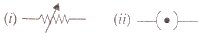Solution :
(i) Variable resistance.
(ii) A closed plug key.

Question 12:
If 20 C of charge pass a point in a circuit in 1 s, what current is flowing ?
Solution :
Given, Q = 20 C, t = 1s
I = ?
We know that:
I = Q/t.
I = 20/1 = 20A.

Question 13:
A current of 4 A flows around a circuit for 10 s. How much charge flows past a point in the circuit in this time ?
Solution :
Given, I = 4amp, C, t = 10s Q = ?
We know that:
I = Q/t.
Q = 4*10 = 40C.

Question 14:
What is the current in a circuit if the charge passing each point is 20 C in 40 s ?
Solution :
Given, Q = 20 C, t=1s
I = ?
We know that:
I = Q/t.
Thus I = 20/40 = 0.5A.

Question 15:
Fill in the following blanks with suitable words :
(a) A current is a flow of……….. For this to happen there must be a………….
(b) Current is measured in……… using an………… placed in……… in a circuit.

Solution :
(a) electrons; closed
(b) amperes; ammeter; series.

Question 16:
(a) Name a device which helps to maintain potential difference across a conductor (say, a bulb).
If a potential difference of 10 V causes a current of 2 A to flow for 1 minute, how much energy is
transferred ?

Solution :
(a) Cell or battery helps to maintain potential difference across a conductor.
(b) Given: p.d. = 10 V, I = 2amp, t = 1 min = 60s.
We know that:
I=Q/t.
Thus, Q=Ixt.
Q = 2×60.
Q = 120 C.
Work done = p.d. x charge moved
Work done = 120x10J
Work done = 1200J.

Question 17:
(a) What is an electric current ? What makes an electric current flow in a wire ?
(b) Define the unit of electric current (or Define ampere).

Solution :
(a) An electric currrent is a flow of electric charges (electrons) through a conductor.
Potential difference between the ends of the wire makes electric current to flow in the wire.
(b) When 1 coulomb of charge flows through any cross-section of a conductor in 1 second, the electric current flowing through it is said to be 1 ampere.

Question 18:
What is an ammeter ? How is it connected in a circuit ? Draw a diagram to illustrate your answer.
Solution :
Ammeter is a device used for the measurement of electric current. It is always connected in series with the circuit in which the current is to be measured.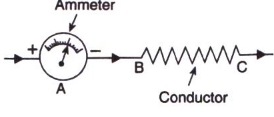Question 19:
(a) Write down the formula which relates electric charge, time and electric current.
(b) A radio set draws a current of 0.36 A for 15 minutes. Calculate the amount of electric charge that flows through the circuit.

Solution :
(a). Work done = Potential difference x charge moved.
(b). I = 0.36A, t = 15min = 900seconds.
Q = Ixt
= 0.36×900
= 324C.

Question 20:
Why should the resistance of :
(a) an ammeter be very small ?
(b) a voltmeter be very large ?

Solution :
(a) The resistance of an ammeter should be very small so that it may not change the value of the current flowing in the circuit.
(b) The resistance of a voltmeter should be very large so that it takes a negligible current from the current.

Question 21:
Draw circuit symbols for (a) fixed resistance (b) variable resistance (c) a cell (d) a battery of three cells (e) an open switch (f) a closed switch.
Solution :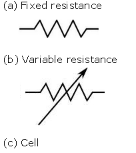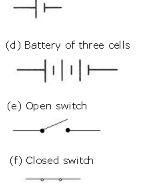Question 22:
What is a circuit diagram ? Draw the labelled diagram of an electric circuit comprising of a cell, a resistor, an ammeter, a voltmeter and a closed switch (or closed plug key). Which of the two has a large resistance : an ammeter or a voltmeter ?
Solution :
A diagram which indicates how different components in a circuit have been connected by using the electrical symbols for the components is called a circuit diagram.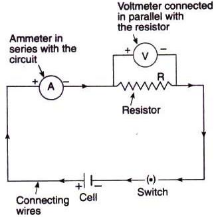A voltmeter has a large resistance.

Question 23:
If the charge on an electron is 1.6 x 10-19 coulombs, how many electrons should pass through a conductor in 1 second to constitute 1 ampere current ?
Solution :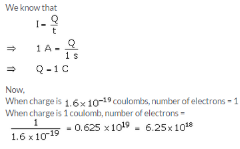Question 24:
The p.d. across a lamp is 12 V. How many joules of electrical energy are changed into heat and light when :
(a) a charge of 1 C passes through it ?
(b) a charge of 5 C passes through it ?
(c) a current of 2 A flows through it for 10 s ?
Solution :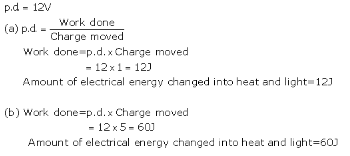(c) I = Q/t

Q= I x t

= 2 x 10 = 20c

Work done = p.d x Charge moved

= 12 x 20 = 2400

Amount of electrical energy changed into heat and light = 2400

Page No:12

Question 25:
In 10 s, a charge of 25 C leaves a battery, and 200 j of energy are delivered to an outside circuit as a result.
(a) What is the p.d. across the battery ?
(b) What current flows from the battery ?

Solution :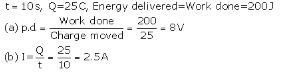Question 26:
(a) Define electric current. What is the SI unit of electric current.
(b) One coulomb of charge flows through any cross-section of a conductor in 1 second. What is the current flowing through the conductor ?
(c) Which instrument is used to measure electric current ? How should it be connected in a circuit ?
(d) What is the conventional direction of the flow of electric current ? How does it differ from the direction of flow of electrons ?
(e) A flash of lightning carries 10 C of charge which flows for 0.01 s. What is the current ? If the voltage is 10 MV, what is the energy ?

Solution :
(a) Electric current is the flow of electric charges (electrons) in a conductor such as a metal wire.
SI unit of electric current is ampere.
(b) 1 ampere.
(c) An ammeter is used to measure electric current. It should be connected in series with the circuit.
(d) Conventional direction of flow of electric current is from positive terminal of a battery to the negative terminal, through the outer circuit. The direction of flow of electrons is opposite to the direction of conventional current, i.e. from negative terminal to positive terminal.

(e) Q = 10C, t= 0.01s

I=Q/t = 10/0.01 = 1000A

p.d = W/Q

W=p.d x Q

=10 x 106 x 10 = 100 x 106 = 100MJ

Energy = work done = 100MJ

Question 32:
A student made an electric circuit shown here to measure the current through two lamps.
(a) Are the lamps in series or parallel ?
(b) The student has made a mistake in this circuit.
What is the mistake ?
(c) Draw a circuit diagram to show the correct way to connect the circuit.
Use the proper circuit symbols in your diagram.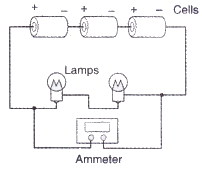Solution :
(a) Lamps are in series.
(b) Student has connected ammeter in parallel with lamps. It should be connected in series.
(c)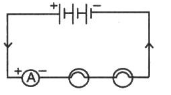Question 33:
Draw a circuit diagram to show how 3 bulbs can be lit from a battery so that 2 bulbs are controlled by the same switch while the third bulb has its own switch.
Solution :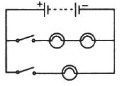Question 34:
An electric heater is connected to the 230 V mains supply. A current of 8 A flows through the heater.
(a) How much charge flows around the circuit each second ?
(b) How much energy is transferred to the heater each second ?

Solution :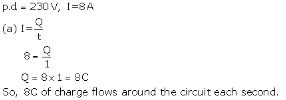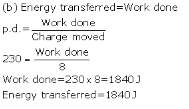Question 35:
How many electrons are flowing per second past a point in a circuit in which there is a current of 5 amp ?
Solution :

I =5A

t= 1s

I= Q/t

Q= I x t = 5 x 1 = 5c

No.  of electron comprising 1.6 x 10-13 C =1

No. of electron comprising 5C = 5/1.6 x 10-19 = 31.25 x 1018

Page No:18

Question 1:
Name the law which relates the current in a conductor to the potential difference across its ends.
Solution :
Ohm’s law

Question 2:
Name the unit of electrical resistance and give its symbol.
Solution :

Unit of electrical resistance os ohm. Its symbol is Ω.

Question 3:
Name the physical quantity whose unit is “ohm”.
Solution :
Electric resistance.

Question 4:
What is the general name of the substances having infinitely high electrical resistance ?
Solution :
Insulators.

Question 5:
Keeping the resistance constant, the potential difference applied across the ends of a component is halved. By how much does the current change ?
Solution :

V= IR

Keeping R constant, V∝I

So, when V is halved, I also become half.

Question 6:
State the factors on which the strength of electric current flowing in a given conductor depends.
Solution :
Strength of electric current flowing in a given conductor depends on
(i) potential difference across the ends of the conductor
(ii) resistance of the conductor.

Question 7:
Which has less electrical resistance : a thin wire or a thick wire (of the same length and same material) ?
Solution :
Thick wire.

Question 8:
Keeping the potential difference constant, the resistance of a circuit is halved. By how much does the current change ?
Solution :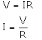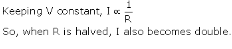Question 9:
A potential difference of 20 volts is applied across the ends of a resistance of 5 ohms. What current will flow in the resistance ?
Solution :
Potential difference, V = 20V
Resistance, R = 5ohms
Current, I = ?
We know that
V = IR
20 = I x 5
I = 20/5 = 4 A

Question 10:
A resistance of 20 ohms has a current of 2 amperes flowing in it. What potential difference is there between its ends ?
Solution :
R = 20ohms
I = 2amp
We know that
V = IR
Thus,
V = 2 x 20
V = 40V

Question 11:
A current of 5 amperes flows through a wire whose ends are at a potential difference of 3 volts. Calculate the resistance of the wire.
Solution :
I = 5amp
p.d., V = 3V
We know that
V=IR
Thus,
3 = 5 x R
R = 3/5 = 0.6 ohm

Question 12:
Fill in the following blank with a suitable word :
Ohm’s law states a relation between potential difference and……………………

Solution :
current.

Question 13:
Distinguish between good conductors, resistors and insulators. Name two good conductors, two resistors and two insulators.
Solution :
Those substances which have very low electrical resistance are called as good conductors. E.g., copper and aluminium.
Those substances which have comparatively high resistance than conductors are known as resistors. E.g., nichrome and manganin.
Those substances which have infinitely high electrical resistance are called insulators. E.g., rubber and wood.

Question 14:
Classify the following into good conductors, resistors and insulators :
Rubber, Mercury, Nichrome, Polythene, Aluminium, Wood, Manganin, Bakelite, Iron, Paper, Thermocol, Metal coin

Solution :
Conductor :- mercury, aluminum, iron, metal coin
Resistor :- manganin, nichrome
Insulator :- rubber, polythene, wood, bakelite, paper, thermocol

Page No:19

Question 15:
What is Ohm’s law ? Explain how it is used to define the unit of resistance.
Solution :
Ohm’s law gives a relationship between current (I) and potential difference (V). According to ohm’s law: At constant temperature, the current flowing through a conductor is directly proportional to the potential difference across its ends.
If I is the current flowing through a conductor and V is the p.d. across its ends, then according to the ohm’s law:

where, R is a constant called "resistance" of the conductor. The unit of resistance is ohm.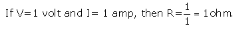Thus, 1 ohm is  the resistance of a conductor such that when a potential differenoe ot 1 volt is applied to its ends, a current of 1 amp flows through it.

Question 16:
(a) What is meant by the “resistance of a conductor” ? Write the relation between resistance, potential
difference and current.
(b) When a 12 V battery is connected across an unknown resistor, there is a current of 2.5 mA in the circuit. Calculate the value of the resistance of the resistor.

Solution :
(a) The property of a conductor due to which it opposes the flow of current through it is called resistance of the conductor.
Work done = Potential difference x charge moved.
(b) V = 12volt, I=2.5 x 10-3 A
We know that
V=IR
R=V/I
R=12/(2.5X10-3)
R=4.8X103ohm = 4800 ohm.

Question 17:
(a) Define the unit of resistance (or Define the unit “ohm”).
What happens to the resistance as the conductor is made thinner ?
Keeping the potential difference constant, the resistance of a circuit is doubled. By how much does the current change ?

Solution :
(a) 1 ohm is the resistance of a conductor such that when a potential difference of 1 volt is applied to its ends, a current of 1ampere flows through it.
(b) Its resistance will increase.
(c)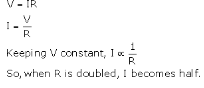Question 18:
(a) Why do electricians wear rubber hand gloves while working with electricity ?
(b) What p.d. is needed to send a current of 6 A through an electrical appliance having a resistance of 40 Ω ?

Solution :
(a) Electricians wear rubber hand gloves while working with electricity because rubber is an insulator and protects them from electric shocks.
(b) I=6amp, R=40ohm
We know that
V=IR
V = 6 x 40 = 240 V.

Question 19:
An electric circuit consisting of a 0.5 m long nichrome wire XY, an ammeter, a voltmeter, four cells of 1.5 V each and a plug key was set up.
(i) Draw a diagram of this electric circuit to study the relation between the potential difference maintained between the points ‘X’ and ‘Y’ and the electric current flowing through XY.
(ii) Following graph was plotted between V and I values :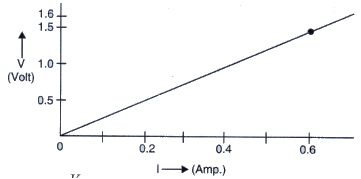(iii) What is the resistance of the wire ?
Solution :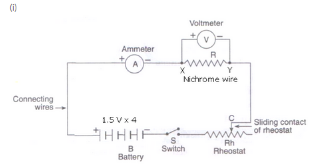(ii) Since the graph is a straight line passing through the origin so current is directly proportional to the potential difference. Hence, die ratior V/I remains constant.

From graoh, when V = 1.5 volt 1 = 0.6 amp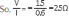For p.d 0.8V, 1.2v and 1.6V. the value of V/I ratio remains the same i.e., 2.5 ohm

The resistance of the wire is. equal to the ratio of potential difference applied and the currrent passing through it.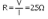Question 20:
(a) What is the ratio of potential difference and current known as ?
(b) The values of potential difference V applied across a resistor and the corresponding values of current I
flowing in the resistor are given below :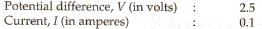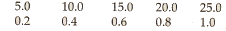Plot a graph between V and I, and calculate the resistance of the resistor.

(c) Name the law which is illustrated by the above V-I graph.
(d) Write down the formula which states the relation between potential difference, current and resistance,
(e) The potential difference between the terminals of an electric iron is 240 V and the current is 5.0 A. What is the resistance of the electric iron ?

Solution :
(a) The ratio of potential difference and current is known as resistance.
(c) Ohm’s law
(d) Potential difference = Current x Resistane
(e) V = 240 volt, I = 5amp
We know that
V = IR
240 = 5 x R
R = 240/5 = 48 ohm.
PAGE 30:

Question 30:
An electric room heater draws a current of 2.4 A from the 120 V supply line. What current will this room heater draw when connected to 240 V supply line ?
Solution :
In first case,
I = 2.4 amp, V = 120 volt
V=IR
120 = 2.4 x R
R = 120/2.4 = 50 ohm
In second case,
V = 240 volt, R = 50 ohm
V = IR
240 = I x 50
I = 4.8 amp.

Question 31:
Name the electrical property of a material whose symbol is “omega”.
Solution :
Resistance.

Question 32:
The graph between V and 1 for a conductor is a straight line passing through the origin.
Which law is illustrated by such a graph ?
What should remain constant in a statement of this law ?

Solution :
(a) Ohm’s law
(b) Temperature.

Question 33:
A p.d. of 10 V is needed to make a current of 0.02 A flow through a wire. What p.d. is needed to make a current of 250 mA flow through the same wire ?
Solution :
In first case,
I = 0.02 amp, V = 10 volt
V = IR
10 = 0.02 x R
R = 10/0.02 = 500 ohm
In second case,
I = 250 x 10-3 amp, R = 500 ohm
V = IR
V = 250 x 10-3 x 500
V = 125 volt.

Question 34:
A current of 200 mA flows through a 4 kΩ resistor. What is the p.d. across the resistor ?
Solution :
I = 200mA = 0.2 A
R = 4 x 103ohm = 4000 ohm
We know that
V = IR
V = 0.2 x 4000
V = 800 volt.

Page No:27

Question 22:
How does the resistance of a wire change when :
(i) its length is tripled ?
(ii) its diameter is tripled ?
(iii) its material is changed to one whose resistivity is three times ?

Solution :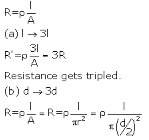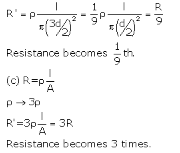Question 23:
Calculate the area of cross-section of a wire if its length is 1.0 m, its resistance is 23 Ω and the resistivity of the material of the wire is 1.84 x 10-6 Ωm.
Solution :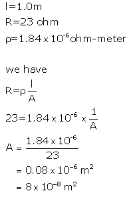Question 24:
(a) Define resistivity. Write an expression for the resistivity of a substance. Give the meaning of each symbol
which occurs in it.
(b) State the SI unit of resistivity.
(c) Distinguish between resistance and resistivity.
(d) Name two factors on which the resistivity of a substance depends and two factors on which it does not depend.
(e) The resistance of a metal wire of length 1 m is 26 Ω at 20°C. If the diameter of the wire is 0.3 mm, what will be the resistivity of the metal at that temperature ?

Solution :

(a) Resistivity,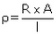where, R is the resistance of the conductor A is the area of cross-section of the conductor I is the length of the conductor.

(b) ohm-meter
1. Resistance is the property of the conductor. while resistivity is the property of the material of the conductor.
2. Resistance of a conductor is the opposition to the flow of electric current through it. Resistivity of a substance is the opposition to the flow of electric current by a rod of that substance which is 1m2  in cross section.
3.Resistance of a conductor depends on length thickness, nature ot material and temperature ot the conductor: while resistvity of substance depends on the nature of the substance and temperature
(d) Resistivity ot a substance depends on the nature of the substance and its temperature. It does not depend on the length or thickness of the conductor.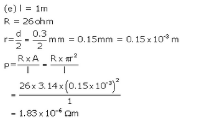Question 33:
A piece of wire of resistance 20 Ω is drawn out so that its length is increased to twice its original length. Calculate the resistance of the wire in the new situation.
Solution :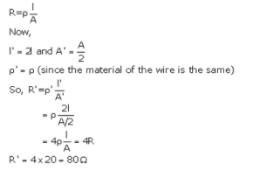Question 34:
The electrical resistivities of three materials P, Q and R are given below :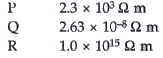Which material will you use for making (a) electric wires (b) handle for soldering iron, and (c) solar cells ? Give reasons for your choices.
Solution :
(a) Material Q with resistivity 2.63 X 10-8 ohm-m can be used for making electric wires because it has very low resistivity.
(b) Material R with resistivity 1.0 X 1015 ohm-m can be used for making handle of soldering iron because it has very high resistivity.
(c) Material P with resistivity 2.3 X 103 ohm-m can be used for making solar cell because it is a semiconductor.

Question 35:
The electrical resistivities of four materials A, B, C and D are given below :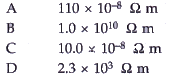Which material is : (a) good conductor (b) resistor (c) insulator, and (d) semiconductor ?
Solution :
(a) Good conductor = C (10 x 10-8ohm-m)
(b) Resistor = A (110 x 10-8 ohm-m)
(c) Insulator= B (1 x 1010 ohm-m)
(d) Semiconductor= D (2.3 x 103 ohm-m)

Question 36:
The electrical resistivities of five substances A, B, C, D and E are given below :
A 5.20 x l0-8 Ω m

Solution :
(a) E is best conductor of electricity due to its least electrical resistivity.
(b) C, because its resistivity is lesser than that of A.
(c) B, because it has the highest electrical resistivity.
(d) C and E, because of their low electrical resistivities.

Page No:37

Question 1:
Give the law of combination of resistances in series.
Solution :
According to the law of combination of resistances in series, the combined resistance of any number of resistances connected in series is equal to the sum of the individual resistances.

Question 2:
If five resistances, each of value 0.2 ohm, are connected in series, what will be the resultant resistance ?
Solution :
As per the law of combination of resistances in series,
R=R1+ R2+ R3+ R4+ R5
R=0.2+0.2+0.2+0.2+0.2=1ohm.

Question 3:
State the law of combination of resistances in parallel.
Solution :
According to the law of combination of resistance in parallel, the reciprocal of the combined resistance of a number of resistances connected in parallel is equal to the sum of the reciprocals of all the individual resistances.

Question 4:
If 3 resistances of 3 ohm each are connected in parallel, what will be their total resistance ?
Solution :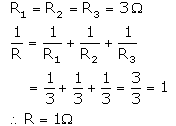Question 5:
How should the two resistances of 2 ohms each be connencted so as to produce an equivalent resistance of 1 ohm ?
Solution :
Since the resultant resistance is less than the individual resistances, so the resistances should be connected in parallel.

Question 6:
Two resistances X and Y are connected turn by turn : (i) in parallel, and (ii) in series. In which case the resultant resistance will be less than either of the individual resistances ?
Solution :
In case of parallel combination, the resultant resistance will be less than either of the individual resistances.

Question 7:
What possible values of resultant resistance one can get by combining two resistances, one of value 2 ohm and the other 6 ohm ?
Solution :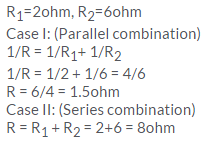Offer running on EduRev: Apply code STAYHOME200 to get INR 200 off on our premium plan EduRev Infinity!

61 docs

,

,

,

,

,

,

,

,

,

,

,

,

,

,

,

,

,

,

,

,

,

,

,

,

;